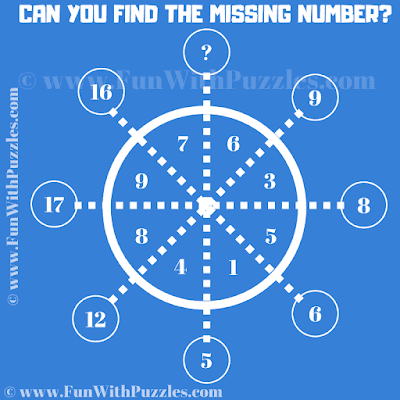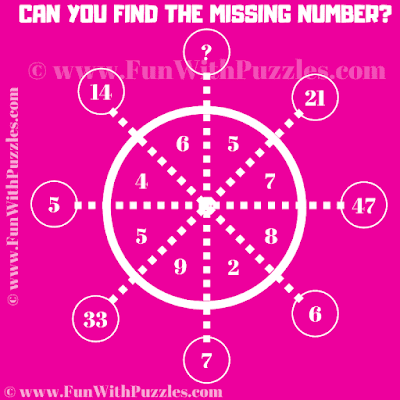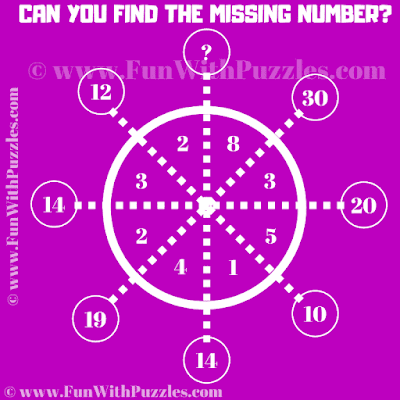These are very interesting number puzzles in which your challenge is to find the missing number in the Circles. In these puzzles, there are some numbers inside the big Circle and also there are numbers inside the small Circles. These number relates to each other with some logical pattern or mathematical formula. Your challenge is to find the logical reasoning which relates these numbers in the Circles. Once you are able to find the logic in these number puzzles, find the value of the missing number which will replace the question mark.
Answers to these puzzles will be provided later. Please write down your answer in the comments along with the logical reasoning used to solve each number puzzle.1. Can you find the missing number in Circle?2. Can you find the missing number in Circle?3. Can you find the missing number in Circle?4. Can you find the missing number in Circle?5. Can you find the missing number in Circle?

The answer to this "....", can be viewed by clicking on the answer button.

Remember the notations given in the below puzzle solution image. The logical reasoning used in these puzzles is explained using this puzzle solution image.

1. 13
B = A1+B2

2. 18
B = 2*A

3.

4.

5.